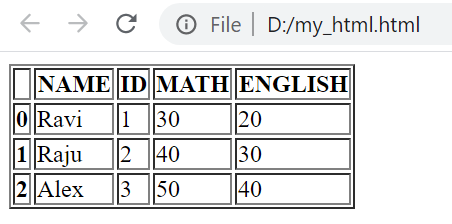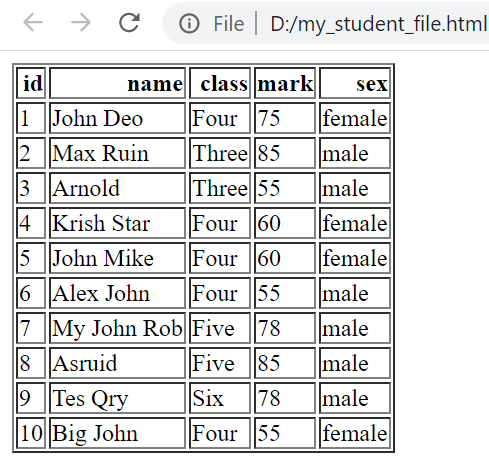# to_html() : DataFrame to html

We will save a DataFrame to .html ( web page ) file. We will create one DataFrame by using a dictionary. From DataFrame we will create the HTML table.
``````import pandas as pd
my_dict={
'NAME':['Ravi','Raju','Alex'],
'ID':[1,2,3],'MATH':[30,40,50],
'ENGLISH':[20,30,41]
}
my_data = pd.DataFrame(data=my_dict)
my_data.to_html('D:\my_html.html')``````Python Pandas html output from DataFrame & using MySQL sample table as source by to_html()

By default we will have index as left most column. We can remove index by using option index=False
``my_data.to_html('D:\my_html.html',index=False)``

## Storing Path

We kept the file in D drive ( root )
``my_data.to_html('D:\my_file.html')``
Inside data directory
``my_data.to_html('D:\data\my_file.html')``

## Options

`buf`: Buffer to write.
`columns` : Default value is None, write all columns. The sub list of columns to generate.
`col_space`: Optional, css width in pixel. Output <th style="min-width: 20px;">
`header`: Default value is True,bool Optional. To print column headers (True ) or not
`index`: Default value is True, bool Optional. To print index row ( True ) or not
`na_rep`: How to handle NaN enteries.
`formatters` : Function to apply for column elements for formatting.
`float_format` : Formatter function for floats.
`sparsify`: To print hierarchical index
`index_names` : Bool , optional, default True. Print name of Indexes
`justify`: Justify column labels. Values are left,right,center,justify,justify-all,start,end,inherit,match-parent,initial,unset.
`max_rows`: int , Optional. Maximum number of rows to display.
`max_cols`: int , Optional. Maximum number of coloumns to display.
`show_dimensions`: bool, default False.
`decimal`: Decimal separator. Example , is used in Europe.
`bold_rows`: Bool, default True, mark row labels bold. `classes`: (HTML ) Class to add to table for style.
`escape` : Bool,default True. Converts < , > and &
`notebook` : Bool, the output is for IPython Notebook.
`border` : int, border width included in <table> tag `table_id` : A css id for the html table.
`render_links` : Default False. Converts URL to Link
`encoding` :str,encoding, default 'utf-8'

Output : String or None based on buf setting.

## Example

``````import pandas as pd
df=pd.read_excel("E:\\data\\student.xlsx") # Path of the file.
l1=['id','name','mark'] # List of columns to show in html table
df.to_html('E:\\data\\my_html.html',max_rows=3,
index=False,justify='left',columns=l1)``````

## na_rep Blank data

How to handle if data is blank, we can use na_rep='*'
``my_data.to_html('D:\my_file.html',na_rep='*')``

## Storing part of the data

We can filter the DataFrame and then save the rows in html file. For this we will use our test.csv file as we have more rows.
Now let us store only two columns, class and name
``````import pandas as pd
df=my_data.loc[:,['class','name']]
my_data = pd.DataFrame(data=df)
my_data.to_html('my_file.html',index=False)``````
We can use various other filters to manage the data and store in html file. You can rad more on filters sections.

## Data from MySQL table to html file

Read on how to connect to MySQL database and then collected the records of student table by using read_sql() to a DataFrame. Finally we will save them in an html file using to_html().
``````import mysql.connector
import pandas as pd
my_connect = mysql.connector.connect(
host="localhost",
user="root",
passwd="*****",
database="my_tutorial"
)
####### end of connection ####
sql="SELECT * FROM student limit 0,5"
my_data.to_html('D:\my_student_file.html',index=False)``````

## Using SQLAlchemy

We will collect records from our sample student table in MySQL database and display as html using to_html().
Collect SQL dump of sample student table below.
Read more on MySQL with SQLAlchemy connection. you can add path if you want the file ( with html tags )to be created with the ( sample is given above )
``````import pandas as pd
from sqlalchemy import create_engine
my_conn = create_engine("mysql+mysqldb://userid:pw@localhost/my_db")
sql="SELECT * FROM student LIMIT 0,10 "
df.to_html('D:\\my_data\\my_html.html')``````

## From Excel file to HTML output

In above code we have created one DataFrame by taking data from a MySQL database table. We can create DataFrame by using any excel data or by using any csv file or from any other sources. ( check here to create a DataFrame from 8 different sources )
Once a DataFrame is created, then using that we can create html output by using to_html(). Here is one example to read one Excel file to a DataFrame and generate the string, you can explore other sources to create a DataFrame and finally generate html tags / file.
``````df=pd.read_excel("D:\\my_data\\student.xlsx") # Path of the file.
df.to_html('D:\\my_data\\my_html.html')``````
``````df=pd.read_csv("D:\\my_data\\student.csv") # change the path
df.to_html('D:\\my_data\\my_html.html')``````## Adding style using classes option

``````import pandas as pd
df=pd.read_excel("E:\\data\\student.xlsx") # Path of the file.
df.to_html('E:\\data\\my_html.html',classes='center') # File created``````
Above code will create my_html.html file at the given path by adding the class=center to center allign the table. The top part of the html source is here.
``````<table border="1" class="dataframe center">
<tr style="text-align: right;">
-----
-----``````
As the class is added to html code, we can define the html class in our style properties. Keep this code in your page CSS or inside HEAD tag. Here is one example.
``````<style>.center {
margin-left: auto;
margin-right: auto;
}</style>``````
To print the html output to console.
``print(df.to_html(classes='center'))``

Subscribe to our YouTube Channel here

## Subscribe

* indicates required
Subscribe to plus2netplus2net.com